Business Mathematics Mock Test - 2

# Business Mathematics Mock Test - 2

Test Description

## 100 Questions MCQ Test Mock Tests & Past Year Papers for CA Foundation | Business Mathematics Mock Test - 2

Business Mathematics Mock Test - 2 for CA Foundation 2023 is part of Mock Tests & Past Year Papers for CA Foundation preparation. The Business Mathematics Mock Test - 2 questions and answers have been prepared according to the CA Foundation exam syllabus.The Business Mathematics Mock Test - 2 MCQs are made for CA Foundation 2023 Exam. Find important definitions, questions, notes, meanings, examples, exercises, MCQs and online tests for Business Mathematics Mock Test - 2 below.
Solutions of Business Mathematics Mock Test - 2 questions in English are available as part of our Mock Tests & Past Year Papers for CA Foundation for CA Foundation & Business Mathematics Mock Test - 2 solutions in Hindi for Mock Tests & Past Year Papers for CA Foundation course. Download more important topics, notes, lectures and mock test series for CA Foundation Exam by signing up for free. Attempt Business Mathematics Mock Test - 2 | 100 questions in 100 minutes | Mock test for CA Foundation preparation | Free important questions MCQ to study Mock Tests & Past Year Papers for CA Foundation for CA Foundation Exam | Download free PDF with solutions
 1 Crore+ students have signed up on EduRev. Have you?
Business Mathematics Mock Test - 2 - Question 1

### An object of a set is called _____________

Detailed Solution for Business Mathematics Mock Test - 2 - Question 1

An object of a set is called an element.
Elements of a set are represented within curly braces{}.

Business Mathematics Mock Test - 2 - Question 2

### If R is a relation from a finite set A having m elements to a finite set B having n elements, then the number of relations from A to B is

Detailed Solution for Business Mathematics Mock Test - 2 - Question 2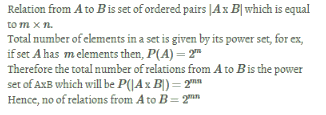Business Mathematics Mock Test - 2 - Question 3

### Which term of the AP, 21,42,63,84,... is 210?

Detailed Solution for Business Mathematics Mock Test - 2 - Question 3

First term of the AP, a=21
Common difference d=21
nth term is 210
Then,
210=a+(n−1)d
210=21+(n−1)×21
210=21n
Therefore, n=10
Hence, 210 is 10th term of the series.

Business Mathematics Mock Test - 2 - Question 4

If f(x)=3x−7, then what is the value of f(2)?

Detailed Solution for Business Mathematics Mock Test - 2 - Question 4

Given,
f(x)=3x−7
Putting 2 in place of x, we get
f(2)=3×2−7
f(2)=6−7=−1

Business Mathematics Mock Test - 2 - Question 5

What is the reciprocal ratio of 121x: 25x3

Detailed Solution for Business Mathematics Mock Test - 2 - Question 5

The reciprocal ratio of a:b is b:a
∴ reciprocal ratio of 121x3: 25x3 is 25x3:121x3

Business Mathematics Mock Test - 2 - Question 6

If the relation R:A→B, where A={1,2,3} and B={1,3,5} is defined by R={(x,y):x<y,x∈A,y∈B}, then

Detailed Solution for Business Mathematics Mock Test - 2 - Question 6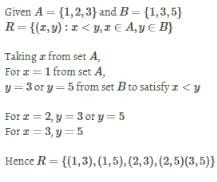Business Mathematics Mock Test - 2 - Question 7

The number of permutations of four letter words obtained from the letters of the word "ARTICLE"is

Detailed Solution for Business Mathematics Mock Test - 2 - Question 7

ARTICLE - total letters : 7
out of them four letters should be
selected to be permutated
7P4
Option : D

Business Mathematics Mock Test - 2 - Question 8

Replace the ? with appropriate alphabet group to complete the given series.

DFST, FSTD, STDF, ?

Detailed Solution for Business Mathematics Mock Test - 2 - Question 8

The first letter in each group is the last letter in the next group, i.e
STDF→TDFS
Hence,option C is the correct answer.

Business Mathematics Mock Test - 2 - Question 9

Student line up in a queue in which Ashish stands fifteenth from the left and Sachin is seventh from the right. If they interchange their places, Sachin would be fifteenth from the right. How many students are there in the queue?

Detailed Solution for Business Mathematics Mock Test - 2 - Question 9

Sachin's new position if 15th from the right as well as left.
Hence, number of students in the queue
=14+1=14
=29
Hence the answer is option C

Business Mathematics Mock Test - 2 - Question 10

Find two numbers such that twice of the first added to the second gives 21, and twice the second added to the first gives 27.

Detailed Solution for Business Mathematics Mock Test - 2 - Question 10

Let the first number is x and second is y
Given twice of first added with second gives 21
∴2x+y=21               ...(1)
And given twice of second added with first gives 27
∴x+2y=27               ...(2)
Multiply (1) by 2
Then 4x+2y=42       ...(3)
Subtract (3) with (2)
Then 3x=15
Or x=5
Put the value of x=5 in (1)
Then 10+y=21
Or y=21−10
Or y=11
Then first number is 5 and second is 11.

Business Mathematics Mock Test - 2 - Question 11

Let A={a,b,c} and B={4,5} Consider a relation R defined from set A to set B then R is subset of

Detailed Solution for Business Mathematics Mock Test - 2 - Question 11

If A and B are two non empty sets, the relation from set A to set B is denoted as A×B

Business Mathematics Mock Test - 2 - Question 12

If P+Q means P is husband of Q, P/Q means P is sister of Q, P∗ Q means P is the son of Q. How is D related to A in D∗B+C/A?

Detailed Solution for Business Mathematics Mock Test - 2 - Question 12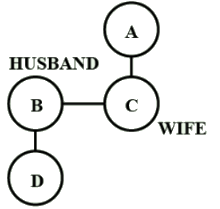P+Q means P is husband of Q
P/Q means P is sister of Q
P∗ Q means P is the son of Q
D∗B means D is the son of B
C/A means C is sister of A
B+C means B is husband of C
So, D is nephew of A.
Hence, Option B is correct.

Business Mathematics Mock Test - 2 - Question 13

Find the ratio of the following :

Q. The speed of cycle is 15km per hour to the speed of scooter 30km per hour.

Detailed Solution for Business Mathematics Mock Test - 2 - Question 13

Speed of cycle which is 15km/hr to the speed of scooter which is 30km/hr=15km/30km=1/2=1:2

Business Mathematics Mock Test - 2 - Question 14

If in a certain language 'mu mit es' means 'who is she' and 'elb mu es' means 'where is she', then what is the code for 'where' in this language?

Detailed Solution for Business Mathematics Mock Test - 2 - Question 14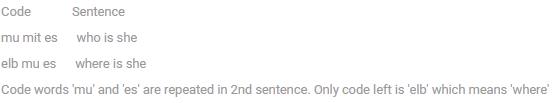Business Mathematics Mock Test - 2 - Question 15

Neelam, who is Deepak's daughter, says to Deepika, "Your mother Rekha is the younger sister of my father who is the third child of Ramlal." How is Ramlal related to Deepika?

Detailed Solution for Business Mathematics Mock Test - 2 - Question 15

Neelam's father is Deepak.
Deepika's mother is Rekha.
Deepak is the third child of Ramlal.
So, Deepak's younger sister Rekha is the daughter of Ramlal.
So, Ramlal is the father of Rekha and the grandfather of her daughter Deepika.
option C.

Business Mathematics Mock Test - 2 - Question 16

What is the reciprocal ratio of 81:121?

Detailed Solution for Business Mathematics Mock Test - 2 - Question 16

The reciprocal ratio of a:ba:b is b:ab:a
∴ reciprocal ratio of 81:121 is 121:81.

Business Mathematics Mock Test - 2 - Question 17

What is the first four terms of the A.P. whose first term is 3 and common difference is 5?

Detailed Solution for Business Mathematics Mock Test - 2 - Question 17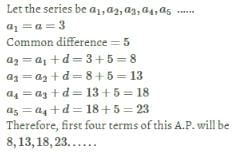Business Mathematics Mock Test - 2 - Question 18

Identify the type of set A={a,b,c}

Detailed Solution for Business Mathematics Mock Test - 2 - Question 18

Here the set A={a,b,c} has finite number of elements. Hence set A is a finite set.

Business Mathematics Mock Test - 2 - Question 19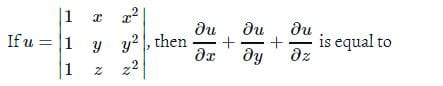Detailed Solution for Business Mathematics Mock Test - 2 - Question 19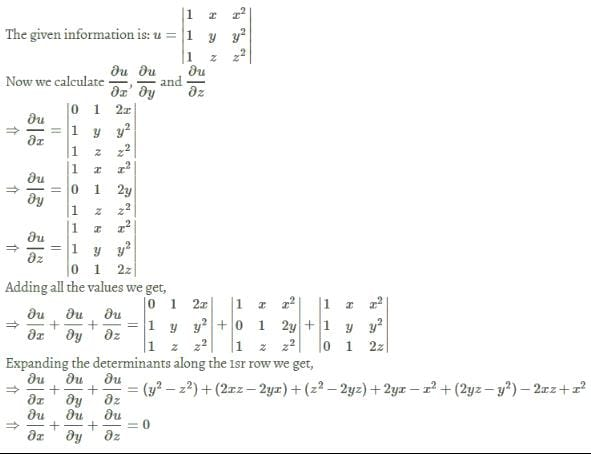Business Mathematics Mock Test - 2 - Question 20

Multiply 5 and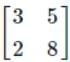. Choose the correct option.

Detailed Solution for Business Mathematics Mock Test - 2 - Question 20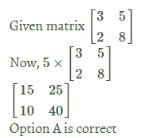Business Mathematics Mock Test - 2 - Question 21

The sum of the present ages of father and his son is 60 years. 6 years ago, father age was five times the age of the son. After six years son's age will be

Detailed Solution for Business Mathematics Mock Test - 2 - Question 21

Let father's age =F
son's age =S
then F+S=60...(1)
and, F−6=5(S−6)
F=5S−24...(2)
From (1),F=60−S
Thus, 60−S=5S−24
84=6S
S=14 years
Present age of son is 14 years.
Hence, after 6 years, son will be 20 years of age

Business Mathematics Mock Test - 2 - Question 22

In how many ways can we select 5 people from a group of 9 people so that a particular person is never to be included

Detailed Solution for Business Mathematics Mock Test - 2 - Question 22

Out of 9 people, one has not to be selected.
Therefore, there are total  8 persons. We have to select 5 among them.
Total number of ways =8C5

Business Mathematics Mock Test - 2 - Question 23

What is 0.9+0.09+0.009+... equal to?

Detailed Solution for Business Mathematics Mock Test - 2 - Question 23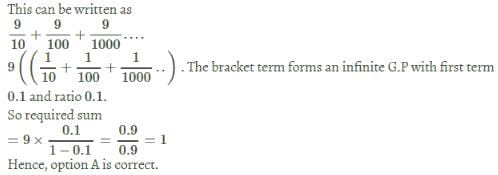Business Mathematics Mock Test - 2 - Question 24

Statements:
1) All grasses are trees.
2) No tree is shrub.

Conclusions:
1) No grasses are shrubs.
2) Some shrubs are grasses.

Detailed Solution for Business Mathematics Mock Test - 2 - Question 24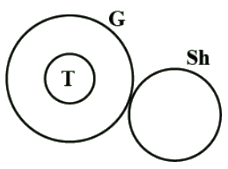G ⇒ Grasses
T⇒Trees
S ⇒Shrubs
Clearly only statement 1 is true.

Business Mathematics Mock Test - 2 - Question 25

chatter : talk :: flutter : ______

Detailed Solution for Business Mathematics Mock Test - 2 - Question 25

To chatter is to talk rapidly, and to flutter is to flap rapidly.

Business Mathematics Mock Test - 2 - Question 26

Find the odd pair out.

Detailed Solution for Business Mathematics Mock Test - 2 - Question 26

Flower is a subset of bouquet
Page is a subset of book....
But Gallon doesn't belong to quintal.
Hence, option A is the correct answer.

Business Mathematics Mock Test - 2 - Question 27

log327 is equal to____

Detailed Solution for Business Mathematics Mock Test - 2 - Question 27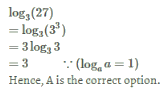Business Mathematics Mock Test - 2 - Question 28

How many words can be formed with the letters of the word ′OMEGA′ such that O and A occupy end places

Detailed Solution for Business Mathematics Mock Test - 2 - Question 28

O_ _ _ _ _A
The three letters can be arranged in 3!=6 while o and a can exchange their places.
Number of ways =3!×2!=12

Business Mathematics Mock Test - 2 - Question 29

Laugh : Joy : : Weep : ?

Detailed Solution for Business Mathematics Mock Test - 2 - Question 29

Laugh indicates joy similarly weep indicates grief

Business Mathematics Mock Test - 2 - Question 30

Four numbers are given below out of which three are alike in some manner and fourth is different. Choose the different number :

Detailed Solution for Business Mathematics Mock Test - 2 - Question 30

All are perfect squares except 168
Hence, option B is the odd one out.

Business Mathematics Mock Test - 2 - Question 31

What is the reciprocal ratio of 21:31?

Detailed Solution for Business Mathematics Mock Test - 2 - Question 31

The reciprocal ratio of a:b is b:a
∴ reciprocal ratio of 21:31 is 31:21

Business Mathematics Mock Test - 2 - Question 32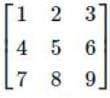The new matrix obtained after adding 2nd row to 3 times 3rd row is

Detailed Solution for Business Mathematics Mock Test - 2 - Question 32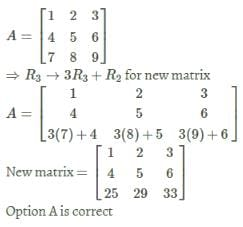Business Mathematics Mock Test - 2 - Question 33

Differentiate with respect to x ex5

Detailed Solution for Business Mathematics Mock Test - 2 - Question 33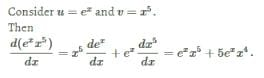Business Mathematics Mock Test - 2 - Question 34

Pointing towards a man in the photograph. Archana said 'He is the son of the only son of my grandmother'. How is man related to Archana?

Detailed Solution for Business Mathematics Mock Test - 2 - Question 34

The given statement says, 'He is the son of the only son of my grandmother'.

Only son of Archana's grandfather means Archana's father

His son is Archana's brother.

Business Mathematics Mock Test - 2 - Question 35

If A,B, C are three matrices such that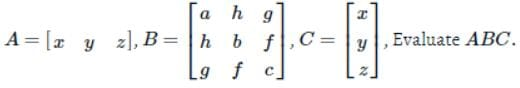Detailed Solution for Business Mathematics Mock Test - 2 - Question 35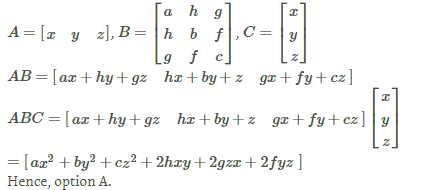Business Mathematics Mock Test - 2 - Question 36

Tiya has Rs.59. She buys a comic book for Rs.32Rs.32. How much money is left with her?

Detailed Solution for Business Mathematics Mock Test - 2 - Question 36

⇒  Tiya has Rs.59.
⇒  Money spend on comic book =Rs.32.
∴  Money left with Tiya =Rs.59−Rs.32=Rs.27.
∴  Money left with Tiya is Rs.27

Business Mathematics Mock Test - 2 - Question 37

What kind of equations are x+y=1 and 3x−2y=4?

Detailed Solution for Business Mathematics Mock Test - 2 - Question 37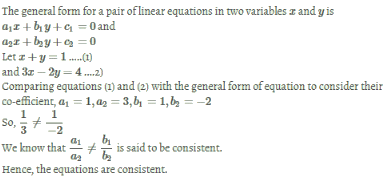Business Mathematics Mock Test - 2 - Question 38

If set P = set M, then ,

Detailed Solution for Business Mathematics Mock Test - 2 - Question 38

If Set P= Set  M, this means they wll have the same elements.
So, the number of elements in the sets will also be the same.
Hence, n(P)=n(M)

Business Mathematics Mock Test - 2 - Question 39

If R is relation from a finite set A having m elements to a finite set B having n elements, then the number of relations from A to B is

Detailed Solution for Business Mathematics Mock Test - 2 - Question 39

A×B  will have mn ordered pairs. Each subset of
A×B will be relation. The number of subsets of a
set consisting of mn elements will be 2mn.
Note : If m=n,then corresponding number will be 2n2.

Business Mathematics Mock Test - 2 - Question 40

If g(x)=x2 and g(w)=25, then find w.

Detailed Solution for Business Mathematics Mock Test - 2 - Question 40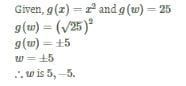Business Mathematics Mock Test - 2 - Question 41

The sum of the series 13−23+33−.........+93=

Detailed Solution for Business Mathematics Mock Test - 2 - Question 41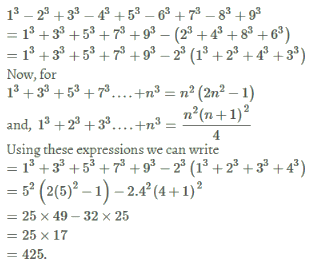Business Mathematics Mock Test - 2 - Question 42

Find the missing letters

3,9,27,81,......

Detailed Solution for Business Mathematics Mock Test - 2 - Question 42

Pttern is 3,3×3,9×3,27×3......
∴ Misssing number = 81×3=243

Business Mathematics Mock Test - 2 - Question 43

In a certain code language, '324' means 'Light is bright', '629' means 'Girl is beautiful' and '4758' means 'I prefer bright clothes'. Which digit means 'Light' in that language?

Detailed Solution for Business Mathematics Mock Test - 2 - Question 43

In the first and second statement, we find that '2' means 'is'. In the first and third statement, we find that '4' means 'bright'. Thus in the first statement, '3' means 'light'.
Hence, option (A) is the correct answer.

Business Mathematics Mock Test - 2 - Question 44

Who amongst P, Q, R, S T and U is the tallest?

I. P is taller than R and T, but not as tall as U, who is taller than Q and S.
II. R is the third in the height in the ascending order and not as tall as U, P and Q, Q being taller than P but not the tallest.

Detailed Solution for Business Mathematics Mock Test - 2 - Question 44

Clearly,
Everyone is shorter than U from statement I. Thus, U is tallest.

Business Mathematics Mock Test - 2 - Question 45

Solve for x:logx125=3?

Detailed Solution for Business Mathematics Mock Test - 2 - Question 45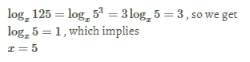Business Mathematics Mock Test - 2 - Question 46

If g(x)=x2g(x)=x2, then calculate g(−2)

Detailed Solution for Business Mathematics Mock Test - 2 - Question 46

Given, g(x)=x2
We have to calculate g(−2)
Putting −2 in place of x, we get
g(−2)=(−2)2=4

Business Mathematics Mock Test - 2 - Question 47

Choose the correct option about conclusion drawn from the statements given below.

Statements:
Some actors are singers.
All the singers are dancers.

Conclusions:
1. Some actors are dancers.
2. No singer is actor.

Detailed Solution for Business Mathematics Mock Test - 2 - Question 47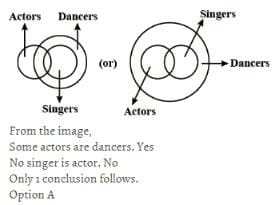Business Mathematics Mock Test - 2 - Question 48

Understanding the code, PITCH = SLWFK, find the correct code language for the word 'BLASTER', from the given alternatives.

Detailed Solution for Business Mathematics Mock Test - 2 - Question 48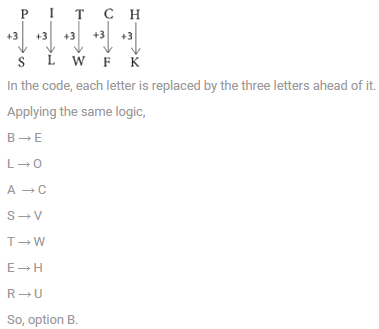Business Mathematics Mock Test - 2 - Question 49

In the following question, there are four options. Three numbers, in these options, are alike in certain manner. Only one number does not fit in. Find the one which doesn't fit.

Detailed Solution for Business Mathematics Mock Test - 2 - Question 49

In all other group of letters except D, third and second letters are consecutive whereas first letter is 3 steps ahead from the second in alphabetic series.
But in D, 3rd and 2nd letters are consecutive whereas 1st and 2nd letters differ by +4.
Hence, the group of letters in D is different from others.

Business Mathematics Mock Test - 2 - Question 50

If log10(x+5)=1, then value of x is equal to

Detailed Solution for Business Mathematics Mock Test - 2 - Question 50

In exponent form
(x+5)=101
∴x=10−5=5

Business Mathematics Mock Test - 2 - Question 51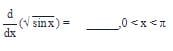Detailed Solution for Business Mathematics Mock Test - 2 - Question 51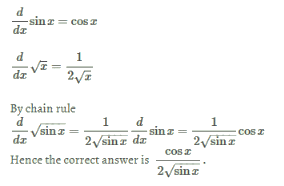Business Mathematics Mock Test - 2 - Question 52

If A =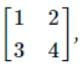then which of the following is not an element of A?

Detailed Solution for Business Mathematics Mock Test - 2 - Question 52

0 is not present in given matrix.
Option A is correct.

Business Mathematics Mock Test - 2 - Question 53

f is a function from set A to set B, Then A is called .........

Detailed Solution for Business Mathematics Mock Test - 2 - Question 53

f:A→B
A is called the domain of the function f:A→B.
B is the range of function.

Business Mathematics Mock Test - 2 - Question 54

If y=tan−1(cot(π/2−x)) then dy/dx=

Detailed Solution for Business Mathematics Mock Test - 2 - Question 54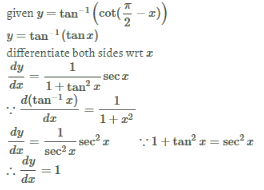Business Mathematics Mock Test - 2 - Question 55

Spot the odd one out.

Detailed Solution for Business Mathematics Mock Test - 2 - Question 55

All others are underground vegetables.

Business Mathematics Mock Test - 2 - Question 56

If air is called water, water is called green, green is called dust, dust is called yellow and yellow is called cloud, which of the following does fish live in ?

Detailed Solution for Business Mathematics Mock Test - 2 - Question 56

We know that fish lives in water, but it is given that water is called green.
Therefore, fish lives in green.
Hence, option C is the correct answer.

Business Mathematics Mock Test - 2 - Question 57

log18 is same as_____

Detailed Solution for Business Mathematics Mock Test - 2 - Question 57

log18
=log6×3
=log6+log3
Hence, A is the correct option.

Business Mathematics Mock Test - 2 - Question 58

Write the following set in the set builder form.

F={I,N,D,A}

Detailed Solution for Business Mathematics Mock Test - 2 - Question 58

Clearly these are letters of the word INDIA.
∴F={x|x is letter of the word INDIA.}

Business Mathematics Mock Test - 2 - Question 59

A graph may be defined as a set of points connected by lines called edges. Every edge connects a pair of points. Thus, a triangle is a graph with 3 edges and 3 points. The degree of a point is the number of edges connected to it. For example, a triangle is agraph with three points of degree 2 each. Consider a graph with 12 points. It is possible to reach any point from any other point through a sequence of edges. The number of edges "e" in the graph must satisfy the condition

Detailed Solution for Business Mathematics Mock Test - 2 - Question 59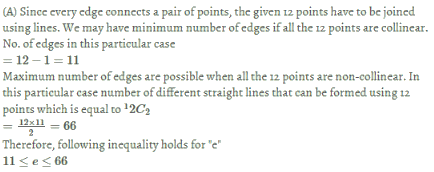Business Mathematics Mock Test - 2 - Question 60

Statements:
1. All pens are pencils.
2. No pencil is a monkey.

Conclusions:
I. No pen is a monkey.
II. some pens are monkeys.
III. All monkeys are pens.

Detailed Solution for Business Mathematics Mock Test - 2 - Question 60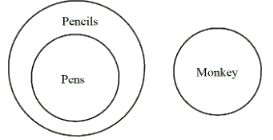From the statements
1. All pens are pencils.
2. No pencil is a monkey.
And From Conclusions

Conclusions:
I. No pen is a monkey.
II. some pens are monkeys.
III. All monkeys are pens.
It is clear that No pen is monkey because from statement it is clear that all pens are pencils
And from Statement two it is clear that no pencil are monkey
Since all pens are pencil's it apply that no pens are monkey

Business Mathematics Mock Test - 2 - Question 61

The mean of 13 observations is 14. If the mean of the first 7 observations is 12 and that of the last 7 observations is 16, then the 7th observation is ___________.

Detailed Solution for Business Mathematics Mock Test - 2 - Question 61

Sum of all observations =13×14=182
Sum of the first 7 observations =7×12=84
Sum of the last 7 observations=7×16=112
Sum of the first 7 observations + Sum of the last 7 observations = sum of total terms + 7th term\$\$
⇒84+112=182+7th term
Therefore, 7th term =196−182=14

Business Mathematics Mock Test - 2 - Question 62

The measure of dispersion is

Detailed Solution for Business Mathematics Mock Test - 2 - Question 62

Mean deviation, standard deviation as well as quartile deviation is the measure of dispersion.
Hence, all of these are measure of dispersion.
(It is well known fact)

Business Mathematics Mock Test - 2 - Question 63

The histogram, given alongside, shows the heights of students (in centimetre) and their numbers.
Use the given histogram to answer the following :

Q. How many students have their height less than 140 cm ?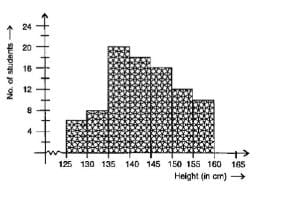Detailed Solution for Business Mathematics Mock Test - 2 - Question 63

Number of students in (125 cm −130 cm)=6
Number of students in (130 cm −135 cm)=8
Number of students in (135 cm −140 cm) =20
So, total number of students less than 140 cm. =20+6+8=34

Business Mathematics Mock Test - 2 - Question 64

If the sum of the mode and mean of the certain frequency distribution in 129 and the median of the observations is 63, mode and mean are respectively

Detailed Solution for Business Mathematics Mock Test - 2 - Question 64

Given, Mode+ Mean = 129 .....(1)
and Median =63,
Also we know, Mode = 3. Median − 2.  Mean
⇒ Mode=3×63−2. Mean =129−2.Mean  ..(2)
Solving (1) and (2) we get, Mean=60 and Mode =69.

Business Mathematics Mock Test - 2 - Question 65

_______ are also used in marketing, monitoring, policy development, bench marking, for lobbying, for the planning of services and for internal research purposes.

Detailed Solution for Business Mathematics Mock Test - 2 - Question 65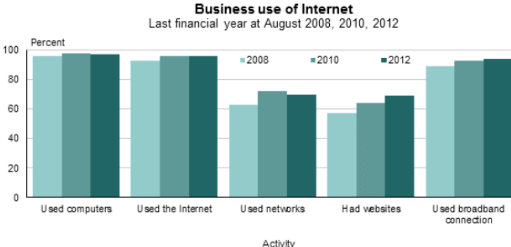Statistics are also used in marketing, monitoring, policy development, bench marking, for lobbying, for the planning of  services and for internal research purposes.
Example: Refer diagram

Business Mathematics Mock Test - 2 - Question 66

The median of a given frequency distribution is found graphically with the help of

Detailed Solution for Business Mathematics Mock Test - 2 - Question 66

The median of a given frequency distribution is found graphically with the help of ogive.
This can be done in two ways:
(i) Presenting the data graphically in the form of 'less than' ogive or 'more than' ogive .
(ii) Presenting the data graphically and simultaneously in the form of 'less than' and 'more than' ogives. in this graph two ogives are drawn together.
Hence, option D is correct.

Business Mathematics Mock Test - 2 - Question 67

If the median of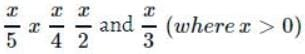is 8 then the value of x would be

Detailed Solution for Business Mathematics Mock Test - 2 - Question 67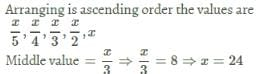Business Mathematics Mock Test - 2 - Question 68

Tally marks are used find

Detailed Solution for Business Mathematics Mock Test - 2 - Question 68

Tally marks are used for counting. They represent frequrncy

Business Mathematics Mock Test - 2 - Question 69

The A.M. of a set of 50 numbers is 38. If two numbers of the set, namely 55 and 45 are discarded, the A.M. of the remaining set of numbers is :

Detailed Solution for Business Mathematics Mock Test - 2 - Question 69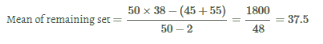Business Mathematics Mock Test - 2 - Question 70

A pictorial representation of data is called

Business Mathematics Mock Test - 2 - Question 71

Which frequency curve is correct for the following histogram?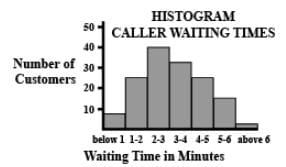Detailed Solution for Business Mathematics Mock Test - 2 - Question 71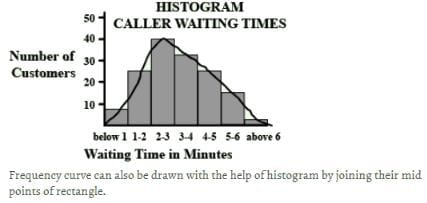Business Mathematics Mock Test - 2 - Question 72

In histogram, the height of rectangle shows _____

Detailed Solution for Business Mathematics Mock Test - 2 - Question 72

A histogram is a display of statistical information that uses rectangles to show the frequency of data items in successive numerical intervals of equal size.
Hence, height of the rectangle represents the frequency of the class.

Business Mathematics Mock Test - 2 - Question 73

If the coefficient of variation and standard deviation of a distribution are 50% and 20 respectively, the its mean is

Detailed Solution for Business Mathematics Mock Test - 2 - Question 73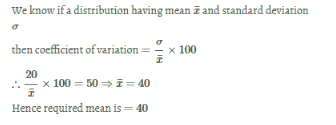Business Mathematics Mock Test - 2 - Question 74

A student got marks in 5 subjects in a monthly test is given below: 2,3,4,5,6.In these obtained marks, 4 is the

Detailed Solution for Business Mathematics Mock Test - 2 - Question 74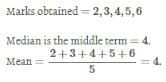Business Mathematics Mock Test - 2 - Question 75

How many people watch T.V. in Chennai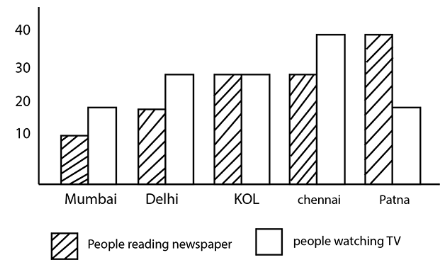Detailed Solution for Business Mathematics Mock Test - 2 - Question 75

Given that bar with lines in the people reading newspaper and the empty bar is the people watching TV.
From the given graph, for the city Chennai, we can observe that the height of the empty bar is 40.
Therefore, Chennai has 40 people who watch TV.

Business Mathematics Mock Test - 2 - Question 76

Find the mean of integers from −4 to 5

Detailed Solution for Business Mathematics Mock Test - 2 - Question 76

integers from −4 to +5 are =−4,−3,−2,−1,0,1,2,3,4,5
sum of all these integers =5
total elements =10
so mean =5/10=0.5

Business Mathematics Mock Test - 2 - Question 77

If the average marks of three batches of 55, 60 and 45 students respectively is 50, 55, 60, then the average marks of all the students is:

Detailed Solution for Business Mathematics Mock Test - 2 - Question 77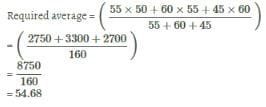Business Mathematics Mock Test - 2 - Question 78

The sum of the squares deviations for 10 observations taken from their mean 50 is 250. The coefficient of variation is

Detailed Solution for Business Mathematics Mock Test - 2 - Question 78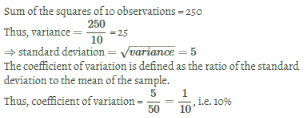Business Mathematics Mock Test - 2 - Question 79

The given bar graph shows the marks obtained by a student in different subjects. The maximum marks of each subject is 100.

Q. In which subject did the student score highest marks?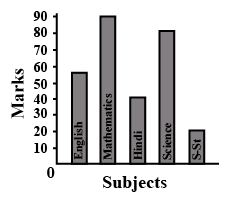Detailed Solution for Business Mathematics Mock Test - 2 - Question 79

Given graph shows the marks obtained in different subjects.
From the graph, the highest marks are 90 which is obtained in Mathematics

Business Mathematics Mock Test - 2 - Question 80

For a certain frequency distribution, the value of Mean is 101 and Median is 100. Find the value of Mode.

Detailed Solution for Business Mathematics Mock Test - 2 - Question 80

We have
Mean =101
Median =100
We know that,
Mean − Mode = 3(Mean − Median)
101− Mode =3(101−100)
100− Mode =3
101−3= Mode
Mode =98

Business Mathematics Mock Test - 2 - Question 81

The sale of salesman in a week are given below in the pie diagram. Study the diagram and answer the following questions, if the total sale due to salesman A is Rs. 18,000.

Q. The salesman with the highest sale is: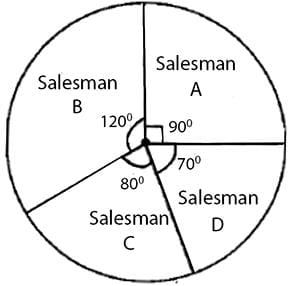Detailed Solution for Business Mathematics Mock Test - 2 - Question 81

In a given pie chart, Salesman B has the greatest angle i.e. 120° when compared to other salesmen.
Also, we know that in a circle, greater the angle of an arc, greater the area.
∴ Salesman B has the highest sale.

Business Mathematics Mock Test - 2 - Question 82

A class teacher has the following absentee record of 40 students of a class for the whole term. Find the mean number of days a student was absent.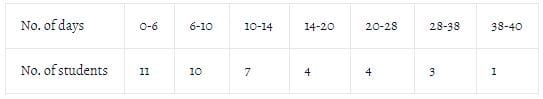Detailed Solution for Business Mathematics Mock Test - 2 - Question 82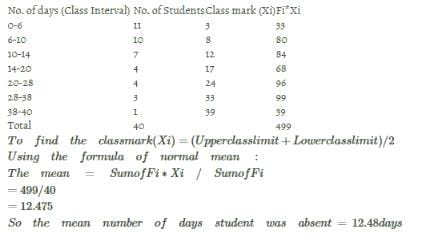Business Mathematics Mock Test - 2 - Question 83

The sum of the squares of deviations of 10 items about mean 50 is 250 .The coefficient of variation is

Detailed Solution for Business Mathematics Mock Test - 2 - Question 83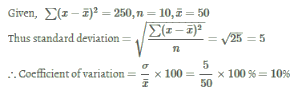Business Mathematics Mock Test - 2 - Question 84

The histogram shows the number of music directors and the number of songs they complete in a year.

Q. Find the number of songs made during the interval 5−6.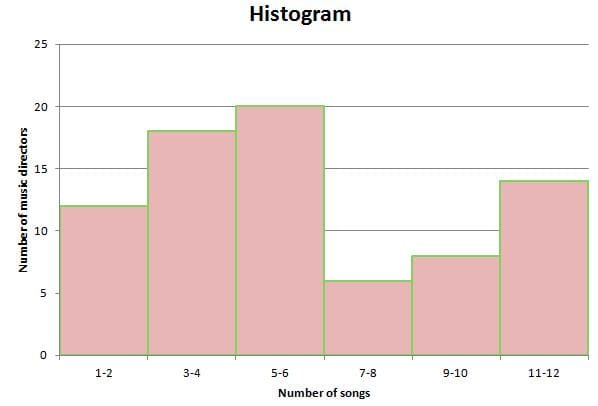Detailed Solution for Business Mathematics Mock Test - 2 - Question 84

The height of the bar represents the number of songs.
So, total of 20 songs made during the interval 5−6.

Business Mathematics Mock Test - 2 - Question 85

The mean square deviation of set of n observations x1, x2,........ xn. about a point c is defined as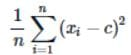The mean square deviation about —2 and 2 are 18 and 10 respectively, then standard deviation of this set of observations is

Detailed Solution for Business Mathematics Mock Test - 2 - Question 85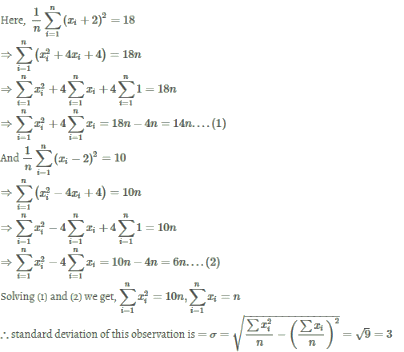Business Mathematics Mock Test - 2 - Question 86

If the difference of Mode and Median of a data is 24, then the difference of Median and Mean is

Detailed Solution for Business Mathematics Mock Test - 2 - Question 86

We have,
Mode=3Median−2Mean
⇒Mode−Median=2(Median−Mean)
⇒24=2(Median−Mean)⇒Median−Mode=12

Business Mathematics Mock Test - 2 - Question 87

A survey was carried out for the purpose of determining the number of children in each family. The results are shown in the table below. Calculate the percentage of families which have more than 2 children.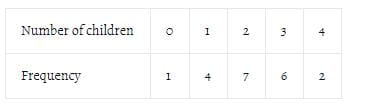Detailed Solution for Business Mathematics Mock Test - 2 - Question 87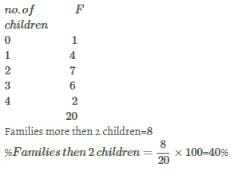Business Mathematics Mock Test - 2 - Question 88

On 13 consecutive days the number of person booked for violating speed limit of 40 km/hr. were as follows
59,52,58,61,68,57,62,50,55,62,53,54,51.

Q. The median number of speed violations per day is

Detailed Solution for Business Mathematics Mock Test - 2 - Question 88

⇒ In the above given data 62 is repeated twice,

⇒ Now we write the data in ascending order.

⇒ 50,51,52,53,54,55,57,58,59,61,62,62,68

⇒ Thus, the measure of (13+1​)/2 = 7th number is median value.

∴ The median number of speed violation per day is 57.

Business Mathematics Mock Test - 2 - Question 89

What are the objectives of measure of dispersion?

Detailed Solution for Business Mathematics Mock Test - 2 - Question 89

Objectives of measure of dispersion:
(i)Reliability of measure of central tendency
(ii)Control of variability
(iii)Helpful in use of further statistical analysis as  in regression, correlation etc

Business Mathematics Mock Test - 2 - Question 90

What is the arithmetic mean of first 16 natural numbers with weights being the number itself?

Detailed Solution for Business Mathematics Mock Test - 2 - Question 90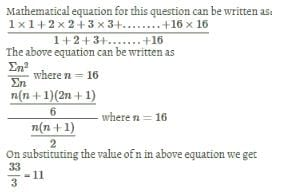Business Mathematics Mock Test - 2 - Question 91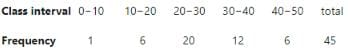Q. Which class has lowest frequency ?

Detailed Solution for Business Mathematics Mock Test - 2 - Question 91

Class 0−10 has lowest frequency i.e. 1.

Business Mathematics Mock Test - 2 - Question 92

There are 30 students in a class. The average age of the first 10 students is 12.5 years. The average age of the remaining 20 students is 13.1 years. What is the average age (in years) of the students of the whole class?

Detailed Solution for Business Mathematics Mock Test - 2 - Question 92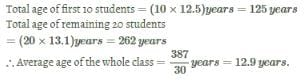Business Mathematics Mock Test - 2 - Question 93

At what subject is the student sharp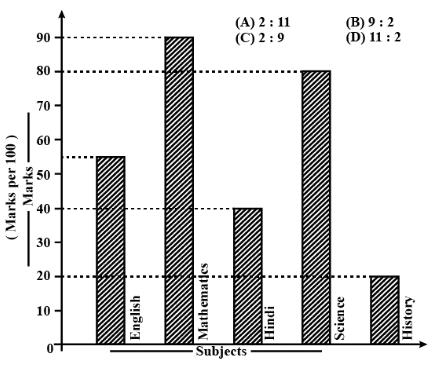Detailed Solution for Business Mathematics Mock Test - 2 - Question 93

Mathematics as its has highest peak

Business Mathematics Mock Test - 2 - Question 94

If M and Mg represents the mean of the raw and grouped data respectively then

Detailed Solution for Business Mathematics Mock Test - 2 - Question 94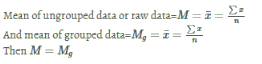Business Mathematics Mock Test - 2 - Question 95

If mean = mode=10. Find median.

Detailed Solution for Business Mathematics Mock Test - 2 - Question 95

We know that mode=3median−2mean
Given that mean=10 and mode=10
Therefore, 10=3median−2(10)
⟹3median=10+20
⟹median=30/3=10

Business Mathematics Mock Test - 2 - Question 96

Find mode of the following data.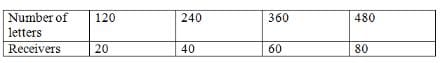Detailed Solution for Business Mathematics Mock Test - 2 - Question 96

Here, the highest frequency of receiving letters is 80.
So, the mode is 480.

Business Mathematics Mock Test - 2 - Question 97

If mean deviation about Mean of a particular data consisting 10 observations is7, then what will be value of mean deviation when each is multiplied by 5?

Detailed Solution for Business Mathematics Mock Test - 2 - Question 97

Suppose original numbers were : x1 , x2, x3, ……, xn
Thus, (x1+x2+x3+…….+xn)/n=10 (by the very definition of mean
After adding 1 to each number, the sum would be : 5× (x1+x2+x3+…….+xn)+n
Thus new mean would be: (5×(x1+x2+x3+…….+xn)+n)/n=5×10+1=51

Business Mathematics Mock Test - 2 - Question 98

Read the shown graph and answer the questions. Highest marks are scored in the subject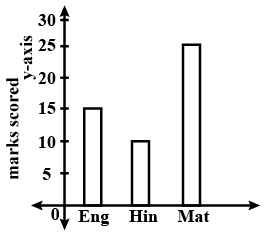Detailed Solution for Business Mathematics Mock Test - 2 - Question 98

Answer:-- Converting the graph into frequency distribution table
Subject   Marks
English  15
Hindi  10
Math 25
The maximum frequency is of maths. i.e. 25.

Business Mathematics Mock Test - 2 - Question 99

Find the mean of the data x,x+a,x+2a,x+3a,...

[(2n+1)terms]

Detailed Solution for Business Mathematics Mock Test - 2 - Question 99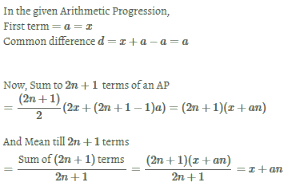Business Mathematics Mock Test - 2 - Question 100

Assertion
If mean & median of an asymmetrical distribution are  58  & 61respectively, then Mode =67.

Reason
For an asymmetrical distribution Mode = 3 Median -2 Mean .

Detailed Solution for Business Mathematics Mock Test - 2 - Question 100

∵ Mode =3 Median −2 Mean
given, Mean=58 Median=61
Thus Mode=3×61−2×58=67
Hence Assertion & Reason bth are correct and Reason is correct explanation  of Assertion.

## Mock Tests & Past Year Papers for CA Foundation

83 docs|19 tests
 Use Code STAYHOME200 and get INR 200 additional OFF Use Coupon Code